# while loop in Python with examples

The "while" loop in Python is used to execute some blocks of code multiple times. I've included all versions of the loop. This article deals with:

## Python while loop syntax

The syntax to use the "while" loop in Python is:

```while expression:
statement(s)```

where "expression" refers to the conditional expression. The expression can also be used with either true or false directly. Or with any conditional expression that must return the result as True or False.

If the conditional expression evaluates to be true, then the program flow goes inside the while loop's block and executes all the statement(s) available in its body. Otherwise, if the condition is evaluated to be false, Then the program flow does not execute its statement(s), and execution of the whole while loop stops.

After executing all the statements available in the body of the while loop, The conditional expression of the loop again gets evaluated. This process continues until the conditional expression is evaluated to be false. Now let's understand while loops practically with the help of the example programs given below.

## Python while loop example

Let's create one of the simplest programs in Python that uses the while loop. That is, the program given below demonstrates while loop in Python:

```i=1
while i <= 10:
print(i)
i = i+1```

Here is the sample output produced by above python program:The dry run of above program goes like:

• Since the first statement of the program is `i=1`. Therefore, initially 1 gets initialized to a variable i. So i=1
• The execution of the second statement, or the execution of the while loop, now begins.
• That is, the condition i <= 10 or 1 <= 10 evaluates to true. Therefore, program flow goes inside the loop.
• Inside the loop, there are two statements. Therefore, both the statements gets executed one by one.
• That is, the statement `print(i)` prints the value of i. That is, 1 get printed on the output.
• And using the second statement, the value of i gets incremented by 1. So i=2 now.
• Because the while loop's statements are all executed. Therefore, the program flow again evaluates the conditional expression of the loop with a new value of i.
• That is, the expression i <= 10 or 2 <= 10 evaluates to true once more.
• Therefore, program flow again goes inside the loop.
• So 2 gets printed on output, and the value of i gets incremented using the second statement of the while loop. Now i=3
• The conditional expression of the while loop again gets evaluated. That is, i <= 10 or 3 <= 10 evaluates to true, the third time.
• This process continues until the condition is evaluated as false.

Important: Don't forget to update (increment or decrease) the loop variable from inside the body of the while loop. Otherwise, the loop would continue its execution forever.

Here is another example of a while loop. This program receives input from the user. And the program continues receiving input from the user until the user enters "learning Python" as input:

```print("Hey! Welcome")
print("What are you doing here ?")
what = input()

while what != "learning python":
what = input("What ?\n")

print("Right.")```

Here are some sample runs of the above while loop example in Python. Here is the initial output that the aforementioned program produced: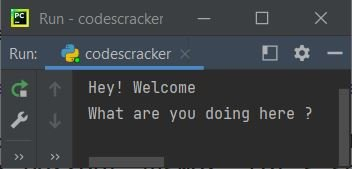Now type your answer, "i don't know," and press the ENTER key to store the input in the "what" variable. Now the input using the "what" variable gets compared with the string "learning Python." If the value of the "what" does not equal this string, then program flow goes inside the loop and receives another input. Here is the output: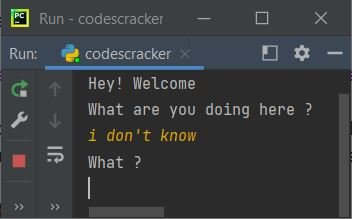Since "i don't know" is not equal to "learning python," Therefore, another input() statement gets executed, which receives another input from the user. Here is another sample run with user input "learning on codescracker":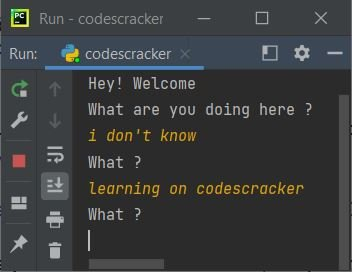Again, the second input is not equal to the string provided in the while loop's conditional expression. As a result, the program will receive input once more. Here is the third sample run. But this time with required input, that is "learning python"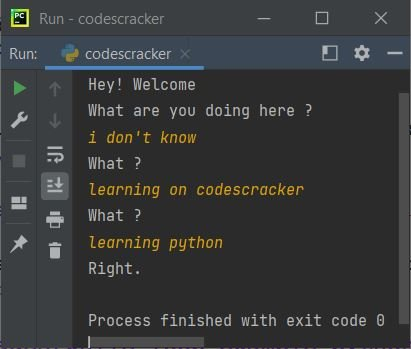### while loop as an infinite loop

If the conditional expression of the while loop always evaluates to true, then the loop goes for infinite execution. For example,

```while True:
statement(s)```

Or

```while 0<10:
statement(s)```

Or

```while "codescracker" == "codescracker":
statement(s)```

etc. Because the first loop employs the "True" keyword, the loop executes indefinitely. In the second while loop's conditional expression, the condition "0<10" always evaluates to true. Therefore, this loop always continues its execution indefinitely. A similar thing goes for the third while loop.

Both expressions, that is, 0<10 and "codescracker" == "codescracker," refer to True indirectly. Because these two conditional expressions are always True.

### How to stop infinite execution of a while loop?

To stop the infinite execution of the while loop, we need to either update the loop variable or use the break keyword from inside the body of the loop. Let's see how.

## while loop with break keyword

The "break" keyword is used to exit from the loop. Or it can be used to skip the remaining execution of the loop.

```i = 1
while True:
val = 2*i
print(val)
i = i+1
if i>10:
break```

The output produced by the above program is shown in the snapshot given below: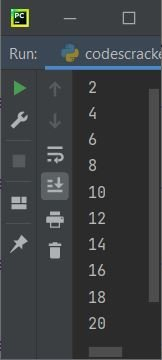The above program of a while loop is created in such a way that the loop continues its execution until the condition of the if, which is i>10, evaluates to true. That is, if the condition evaluates to true, program flow goes inside its block, or if's body, and the break statement gets executed, which stops the remaining execution of the while loop.

## while loop with continue keyword

The "continue" keyword is used to jump for the next iteration, skipping the execution of the remaining statement(s) of the loop's body, if any are available after the keyword.

```i = 9
while i<=20:
i = i+1
if i%2 != 0:
continue
print(i)```

This program prints all the even numbers from 10 to 20 (including both numbers, if even). The snapshot given below shows the sample output produced by the above program: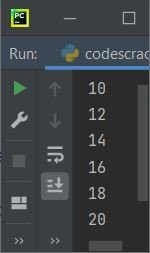## while loop with pass keyword

The "pass" keyword does nothing. It can be used as a placeholder for the code or block of code that has to be implemented in the future.

```mystr = "codescracker"
count = 0

while count < len(mystr):
count = count+1
pass

print("Length of String =", count)```

Here is the output produced by the above program: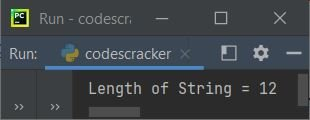However, the above program can also be written without the pass, the output will be the same. Here is the same program without a pass:

```mystr = "codescracker"
count = 0

while count < len(mystr):
count = count+1

print("Length of String =", count)```

I've provided all the details about pass in a separate tutorial. Also compared pass with continue in another separate tutorial.

## while loop with list

The program given below uses list as a conditional expression for the while loop.

```mylist = ['p', 'y', 34.5, 't', 2, 'codescracker', True]

while mylist:
print(mylist.pop())```

The output produced by the above program is shown in the snapshot given below: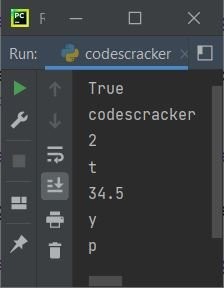In the above program, the pop() method is used to pop or delete a list element from the end.

Here is another program that uses a while loop to implement a list. This program receives the size and elements of the list. And then it prints the list elements back on the output screen, using a while loop:

```print("Enter the Size: ", end="")
tot = int(input())
i = 0
mylist = list()
print("Enter", tot, "Numbers: ", end="")

while i<tot:
val = int(input())
mylist.insert(i, val)
i = i+1

i = 0
print("\n-------The List is-------")

while i<tot:
print(mylist[i], end=" ")
i += 1```

Here is its sample run with user input of 6 as size and 23, 24, 25, 26, 39, and 40 as six elements: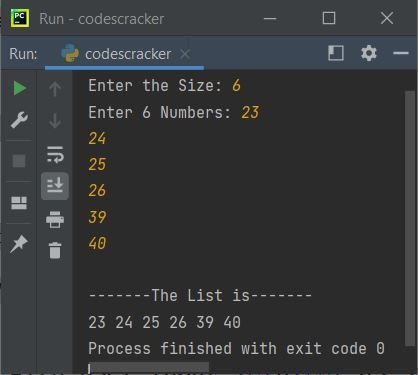The end is used to skip an automatic insertion of newlines using the print() function.

## while loop with else block

The "while" loop with "else" statement is used to execute statement(s) using else after completing the execution of the loop. For example:

```i = 9
while i<=20:
i = i+1
if i%2 != 0:
continue
print(i)
else:
print("All even numbers from 10 to 20 are given above")```

Here is the output produced by this Python program, which shows the use of the else statement with the while loop:### while loop in on a single line

The while loop in Python can also be written in a single line. Here is an example using the loop in a single line:

```i = 1
while i <= 10: print(5 * i); i += 1```

The output produced by the above program should be:

```5
10
15
20
25
30
35
40
45
50```

## Nested while loop

Just like for loop, "while" loop can also be nested inside another "while" loop. Here is an example that demonstrates the use of nested while loops in Python:

```print("Enter any Number: ")
n = int(input())
print("Enter Number of Rows: ")
no_of_rows = int(input())
i = 0
j = 0

while i<no_of_rows:
while j<i+1:
print(n, end=" ")
j = j+1
j = 0
i = i+1
print()```

Here is the initial output produced by the above program:Now enter a number, say 1, and then enter the number of rows, say 5, to print 1 in five rows in such a way that one "1" is at the first row, two "1" is at the second row, three "1" is at the third row, four "1" is at the fourth row, and five "1" is at the fifth row, as shown in the snapshot given below: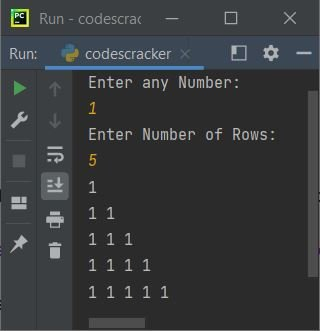#### More Examples

Python Online Test

« Previous Tutorial Next Tutorial »